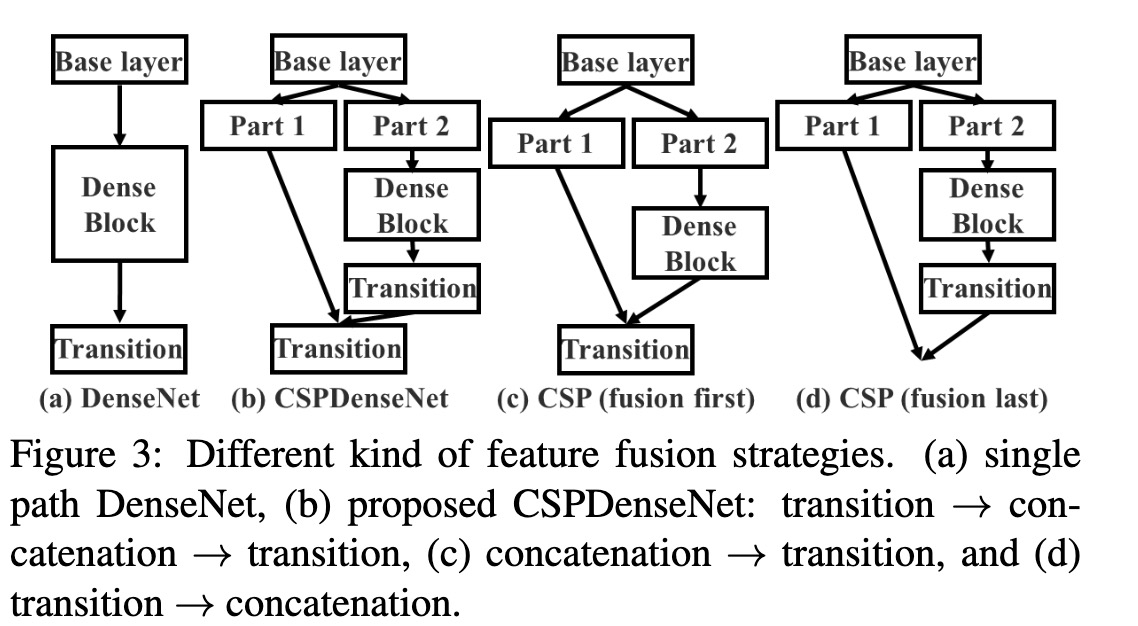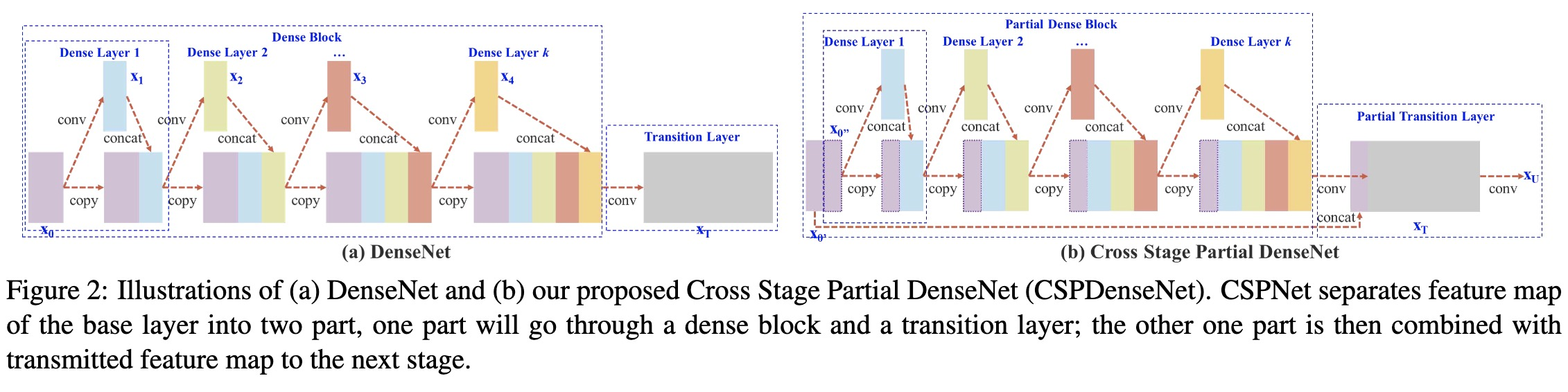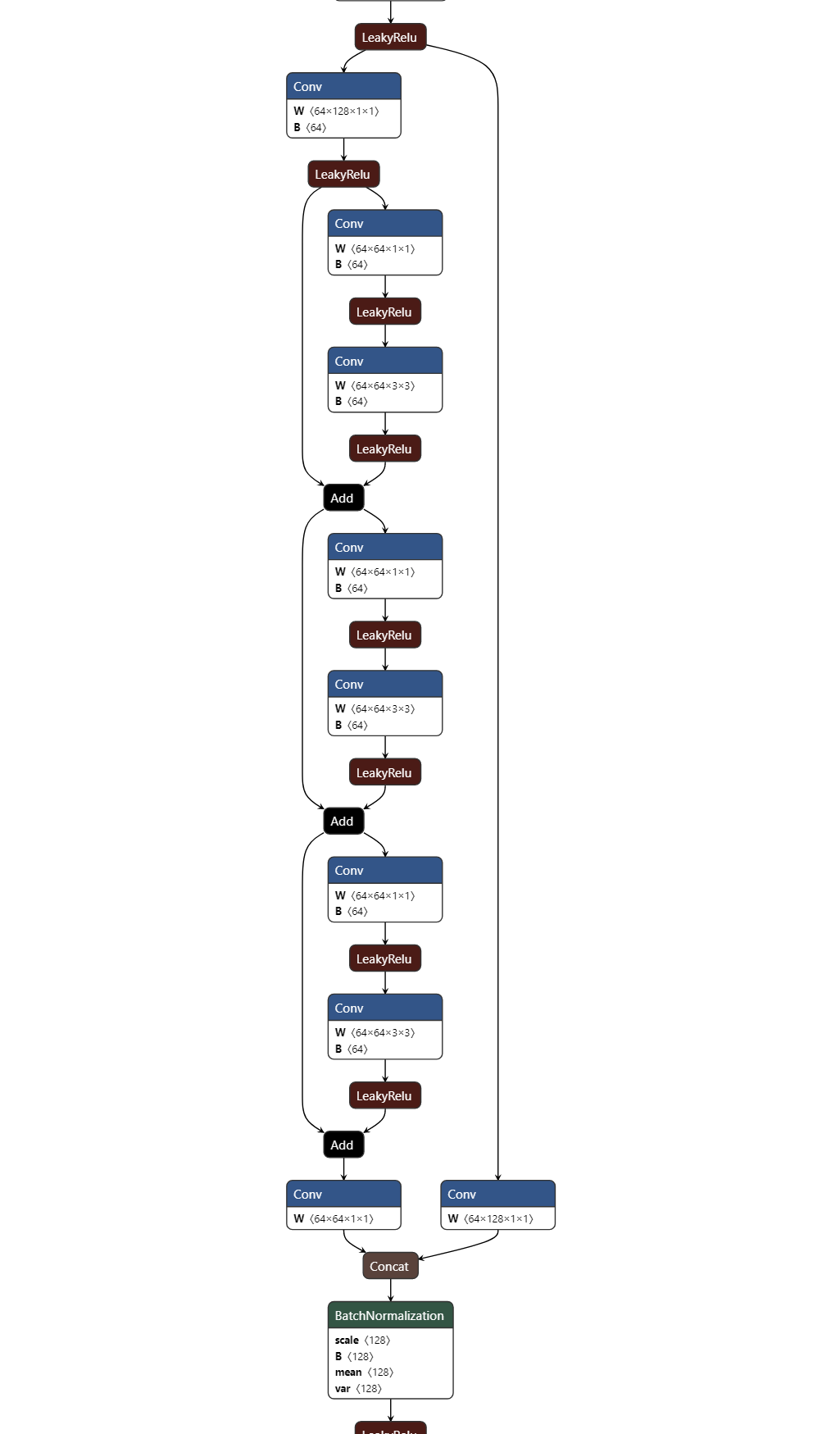CSPNet

CSPNet出至论文：CSPNet: A New Backbone that can Enhance Learning Capability of CNN, 近来yolo-v4,yolo-v5都使用其作为主干网络的结构，其主要用于降低计算量的同时保持甚至提升网络的特征提取能力. 本文主要对其实现作小结，理论分析不深入。

优点

• 增强CNN的学习能力，能够在轻量化的同时保持准确性。
• 降低计算瓶颈
• 降低内存成本

实现a 是原始的densenet 特征融合方式，b 是cspdensenet特征融合方式（trainsition->concatenation->transition），c d分别是作者尝试的另两种融合方式，b是c、d的结合. Fustion First的方式是对两个分支的feature map先进行concatenation操作，这样梯度信息可以被重用。 Fusion Last的方式是对Dense Block所在分支先进性transition操作，然后再进行concatenation， 梯度信息将被截断，因此不会重复使用梯度信息 。 transition为过渡层， 一般是1 * 1卷积.

• 使用Fusion First有助于降低计算代价，但是准确率有显著下降。
• 使用Fusion Last也是极大降低了计算代价，top-1 accuracy仅仅下降了0.1个百分点。
• 同时使用Fusion First和Fusion Last的CSP所采用的融合方式可以在降低计算代价的同时，提升准确率.class Bottleneck(nn.Module):
# Standard bottleneck
def __init__(self, c1, c2, shortcut=True, g=1, e=0.5):  # ch_in, ch_out, shortcut, groups, expansion
super(Bottleneck, self).__init__()
c_ = int(c2 * e)  # hidden channels
self.cv1 = Conv(c1, c_, 1, 1)
self.cv2 = Conv(c_, c2, 3, 1, g=g)
self.add = shortcut and c1 == c2

def forward(self, x):
return x + self.cv2(self.cv1(x)) if self.add else self.cv2(self.cv1(x))

class BottleneckCSP(nn.Module):
# CSP Bottleneck https://github.com/WongKinYiu/CrossStagePartialNetworks
def __init__(self, c1, c2, n=1, shortcut=True, g=1, e=0.5):  # ch_in, ch_out, number, shortcut, groups, expansion
super(BottleneckCSP, self).__init__()
c_ = int(c2 * e)  # hidden channels
self.cv1 = Conv(c1, c_, 1, 1)
self.cv2 = nn.Conv2d(c1, c_, 1, 1, bias=False)
self.cv3 = nn.Conv2d(c_, c_, 1, 1, bias=False)
self.cv4 = Conv(2 * c_, c2, 1, 1)
self.bn = nn.BatchNorm2d(2 * c_)  # applied to cat(cv2, cv3)
self.act = nn.LeakyReLU(0.1, inplace=True)
self.m = nn.Sequential(*[Bottleneck(c_, c_, shortcut, g, e=1.0) for _ in range(n)])

def forward(self, x):
y1 = self.cv3(self.m(self.cv1(x)))
y2 = self.cv2(x)
return self.cv4(self.act(self.bn(torch.cat((y1, y2), dim=1))))

netron 可视化如下: